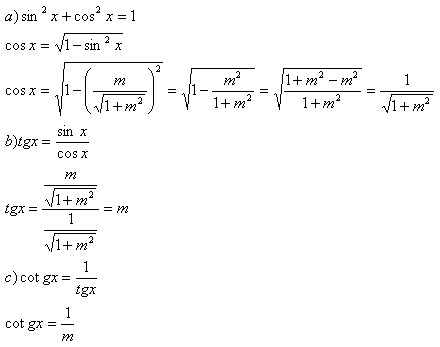sk|cz|

# Trigonometry

## Formulae

To solve following problems you should use formulae from your math textbook.
Most frequently used formulae: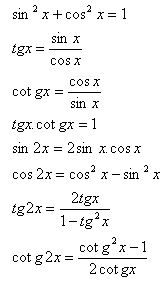If any other formula should be used, it will be mentioned in the set of the task.

1.Find the values of the other trigonometric functions: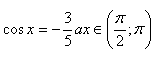Solution: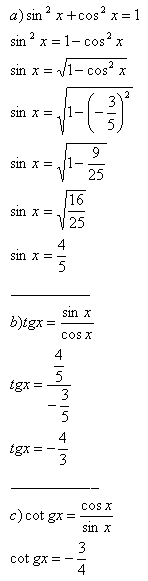2.Find the values of the other trigonometric functions: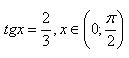Solution: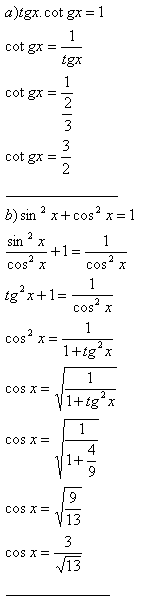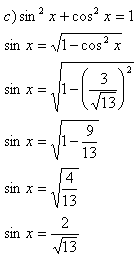3.Find the values of the other trigonometric functions: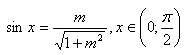Solution: